Students can Download Maths Chapter 5 Geometry Ex 5.1 Questions and Answers, Notes Pdf, Samacheer Kalvi 7th Maths Book Solutions Guide Pdf helps you to revise the complete Tamilnadu State Board New Syllabus and score more marks in your examinations.

## Tamilnadu Samacheer Kalvi 7th Maths Solutions Term 1 Chapter 5 Geometry Ex 5.1

Question 1.
Name the pairs of adjacent angles.
Solution:
(i) ∠ABG and ∠GBC are adjacent angles.
(ii) ∠BCF and ∠FCD are adjacent angles.
(iii) ∠BCF and ∠FCE are adjacent angles.
(iv) ∠FCE and ∠ECD are adjacent angles.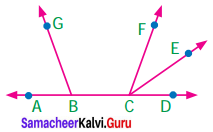Question 2.
Find the angle ∠JIL from the given figure.
Solution:
∠LIK and ∠KIJ are adjacent angles.
∴ ∠JIL = ∠LIK + ∠KIJ
= 38° + 27°
= 65°
∴ ∠JIL = 65°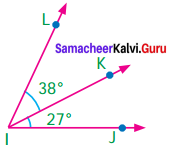Question 3.
Find the angles ∠GEH from the given figure.
Solution: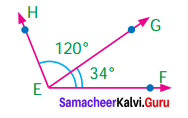∠HEF = ∠HEG + ∠GEF
120° = ∠HEG + 34°
120°- 34° = ∠GEH + 34° – 34°
∠GEH = 86°

Question 4.
Given that AB is a straight line. Calculate the value of x° in the following cases.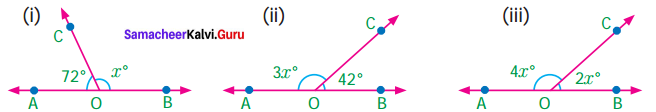Solution:
(i) Since the angles are linear pair ∠AOC + ∠BOC = 180°
72° + x° = 180°
72° + x° – 12° = 180°- 72°
x° = 108°

(ii) Since the angles are linear pair
∠AOC + ∠BOC = 180°
3x + 42° = 180°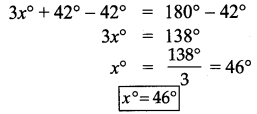(iii) Since the angles are linear pair
∠AOC + ∠BOC = 180°
4x° + 2x° = 180°
6x° = 180°
x° = 180°

Question 5.
One angle of a linear pair is a right angle. What can you say about the other angle?
Solution:
If the angle are linear pair, then their sum is 180°.
Given one angle is right angle ie 90°.
∴ The other angle = 180° -90° = 90°
∴ The other angle also a right angle

Question 6.
If the three angles at a point are in the ratio 1 : 4 : 7, find the value of each angle?
Solution:
We know that the sum of angles at a point is 360°.
Given the three angles are in the ratio 1:4:7.
Let the three angles be 1x, 4x, 7x.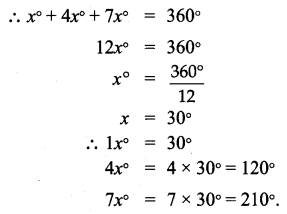∴ The three angles are 30°, 120° and 210°.

Question 7.
Three are six angles at a point. One of them is 45° and the other five angles are all equal. What is the measure of all the five angles.
Solution:
We know that the sum of angles at a point is 360°.
One angle = 45°
Let the equal angles be x° each
∴ x° + x° + x° + x° + x° + 45° = 360°
5x° + 45° – 45° = 360° – 45°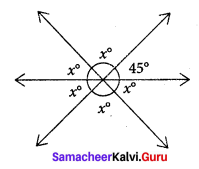5x° = 315°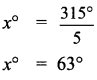∴ Measure of all 5 equal angles = 63°.

Question 8.
In the given figure, identify
(i) Any two pairs of adjacent angles.
(ii) Two pairs of vertically opposite angles.
Solution:
(i) (a) ∠PQT and ∠TOS are adjacent angles.
(b) ∠PQU and ∠UQR are adjacent angles.
(ii) (a) ∠PQT and ∠RQU are vertically opposite angles.
(b) ∠TQR and ∠PQU are vertically opposite angles.

Question 9.
The angles at a point are x°, 2x°, 3x°, 4x° and 5x°. Find the value of the largest angle?
Sum of angles at a point = 360°
∴ x° + 2x° + 3x° + 4x° + 5x° = 360°
15x° = 360°
x° = $$\frac{360^{\circ}}{15}$$
x° = 24°.
∴ The largest angle = 5x°
= 5 × 24° = 120°
The largest angle is 120°

Question 10.
From the given figure, find the missing angle.
Solution:
Lines $$\overleftrightarrow { RP }$$ and $$\overleftrightarrow { SQ }$$ are interesting at ‘O’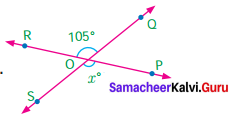∴ Vertically opposite angles are equal.
∴ x = 105°
∴ Missing angle = 105°

Question 11.
Find the angles x° and y° in the figure shown.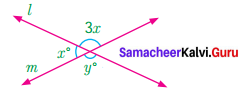Solution:
Consider the line m.
x° and 3x° are linear pair of angles
∴ x° + 3x° = 180°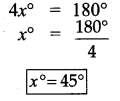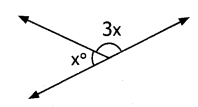x° = 45°
Also lines l and m intersects.
Vertically opposite angles are equal.
ie 3x° = y°
3 × 45° = y°
y = 135°
x° = 15° and
y° = 135°

Question 12.
Using the figure, answer the following questions.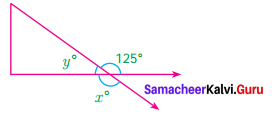(i) What is the measure of angle x°?
(ii) What is the measure of angle y°?
Solution:
From the figure x° and 125° are vertically opposite angles. So they are equal ie
ie x° = 125°
Also y° and 125° are linear pair of angles.
∴ y° + 125° = 180°
y° + 125° – 125° = 180°- 125°
y° = 55°
x° = 125°,
y° = 55°

Question 13.
(i) No common interior, no common arm, no common vertex.
(ii) One common vertex, one common arm, common interior
(iii) One common arm, one common vertex, no common interior.
(iv) One common arm, no common vertex, no common interior.
Solution:
(iii) one common arm, one common vertex, no common interior

Question 14.
In the given figure the angles ∠1 and ∠2 are
(i) Opposite angles
(iii) Linear angles
(iv) Supplementary angles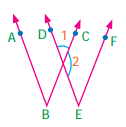Solution:
(iii) Linear pair

Question 15.
Vertically opposite angles are
(i) not equal in measure
(ii) Complementary
(iii) supplementary
(iv) equal in measure
Solution:
(iv) equal in measure

Question 16.
The sum of all angles at a point is
(i) 360°
(ii) 180°
(iii) 90°
(iv) 0°
Solution:
(i) 360°

Question 17.
The measure of ∠BOC is
(i) 90°
(ii) 180°
(iii) 80°
(iv) 100°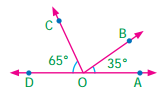Solution:
(iii) 80°